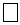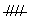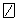www.batmath.it

A Glossary of Mathematical Terms - T

Tally

A method of countign tghat is used to keep tarck of responses in statistical surveys: 4 is talled as ////, or, five is talled asor.

Tessellation

A covering or tiling of the plane with non overlapping shapes. Regular tessellations are made with regular polygons.

Tetragon

A four-sided polygon.

Tilt

The measure of an angle as compared to a horizontal line. (Also what happens when you kick the pinball machine too hard!).

Torus

A 3-D figure formed by rolling a rectangle into a cylinder and bending the cylinder until its bases meet.

See Slide.

Transversal

A line that intersects two other lines.

Trapezoid

A quadrilateral with two parallel sides.

Tree diagram

A diagram used in many circumstances; for example in statistics it represents a systematic way of determining all possible outcomes of an experiment.

Triangle

A three sided polygon.
Right triangle: when one angle is 90°.
Equilateral triangle: all angles are 60°, all sides have equal length.
Isosceles triangle: a triangle with two sides of equal length.
Scalene triangle: a triangle with three different sides and angles.
Acute triangle: a traingle where all angles are less than 90°.
Obtuse triangle: a triangle with one angle that is  more than 90°.

Triangulate

To divide a polygon into triangles.

Tridecagon

A 13-sided polygon.

Trigon

The same as triangle.

Trigonometry

Basic elements: sine, cosine, tangent, cotangent, secant, cosecant.